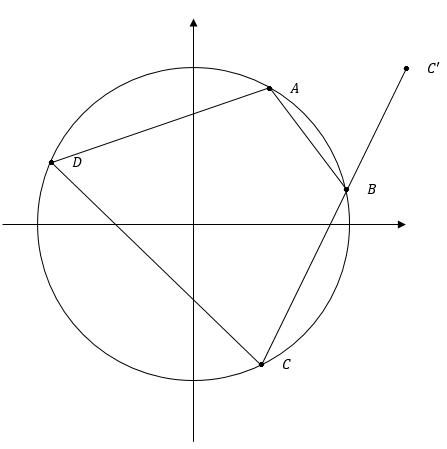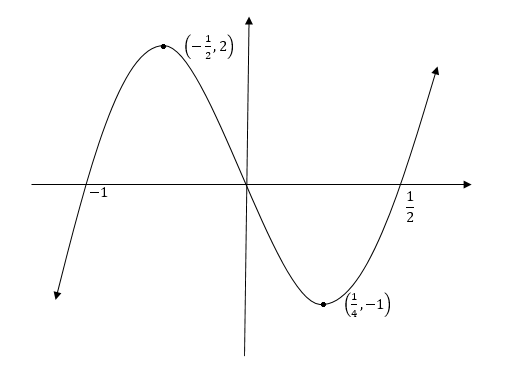# 7 HSC Trial Problems to Test Your Maths Extension 2 Skills

In this post, Head Maths Teacher Oak Ukrit has given you 7 extension 2 questions that you should answer to test if you're HSC ready!The Trials are nearly here. But are you really ready? Really? Let’s find out, Head Maths Teacher, Oak Ukrit, has put together 7 HSC Trial Problems to Test Your Maths Extension 2 Skills.

Let’s find out how ready you really are! Grab your pencil and switch your brain to turbo. Solve!

## Question 1 – Standard Integral problems

a. Evaluate the following integrals

i. $$\int e^{x}sin(x) \ dx$$

ii. $$\int sec \ x \ + sec^{2}x + sec^{3}x+ sec^{4} x \ dx$$

iii. $$\int \frac {2+3x+x^{2}}{x(x^{2}+1)}dx$$

### Answers:

i.

 $$I=\frac{1}{2}e^{x}(sin \ x-cos \ x) + C$$

ii.

 $$I = 2 tan x+ \frac{1}{3} sec^{3}x+ \frac{1}{2} sec \ x \ tan \ x + \frac{3}{2} \mid sex \ x+ tan \ x \mid +C$$

iii.

 $$I=ln \mid \frac{x^{2}}{\sqrt{x}^{2}+1} \mid +3tan^{-1}x+C$$

## Question 2 – Recurrence Formulas and Definite Integral Properties

a. Given that

$$I_{n}= \int \frac{dx}{(x^{2}+a^{2})^{n}}$$

Show that

$$I_{n}= \frac{1}{2a^{2}(n-1)}(\frac{x}{(x^{2}+a^{2})^{n-1}}+(2n-3)I_{n-1})$$

b. Find the value of

$$\int_{0}^{\frac{\pi}{2}}\frac{(sin \ x)^{\frac{\pi}{2}}}{(sin \ x)^{\frac{\pi}{2}}+(cos \ x)^{\frac{\pi}{2}}}dx$$

### Answers:

 b. $$I= \frac{\pi}{4}$$

## Want to Maximise Your Maths Marks?

Suddenly nervous about your Extension 2 HSC but are worried you’re running out of time? Don’t put it off, learn how to boost your Extension 2 HSC Exam marks.## Question 3 – Volumes

a. The region bounded by the circle $$x^{2}+y^{2}=a^{2}$$ is the base of a solid. Cross sections taken perpendicular to the base and parallel to the y-axis are equilateral triangles.

Find the volume of the solid.

b.  A cylindrical hole of constant radius r and height h is bored through the centre of a sphere with radius R.

Find the volume of the solid in terms of h.

c.  The region bounded by the curve $$y=-x^{2}+4x-3$$ and the x-axis is rotated about the line x=3 to form a solid.

Find the volume of the solid.

### Answers:

a.

 $$V=\frac{4}{3}a^{3} \ unit^{3}$$

b.

 $$V=\frac{\pi h^{3}}{6}unit^{3}$$

c.

 $$V=\frac{8 \pi}{3}unit^{3}$$

## Question 4 – Complex Numbers

a. The points A, B, C and D lie on a circle centred at the origin. Let them represent the complex numbers $$z_1,z_2,z_3$$ and $$z_4$$ respectively. CB is produced to ‘.

By considering ∠ABC^’ and ∠ADC, prove that

$$Im(\frac{z_{1}-z_{2}}{z_{2}-z_{3}}\times \frac{z_{3}-z_{4}}{z_{1}-z_{4}})=0$$b.

i. Show that

$$tan5θ = \frac{tan^{5} \ θ-10 \ tan^{3} \ θ+5 \ tan \ θ}{5 \ tan^{4} \ θ-10 \ tan^{2} \ θ+1}$$

ii. By considering the equation $$t^{4}-10t^{2}+5=0$$, show that,

$$tan(\frac{\pi}{5})tan(\frac{2 \pi}{5})-\sqrt5=0$$

## Question 5 – Polynomials

a.  Let α, β and γ be the roots of $$P(x)=x^{3}+x^{2}-3x-2$$

By considering a transformation or otherwise, evaluate:

$$(α+β)^{3}+(α+γ)^{3}+(β+γ)^{3}$$

b. Fully factorise $$P(x) = 12x^{3} -4x^{2}-13x-4$$, given that it is a double root.

### Answer:

a.

 -23

b.

 $$P(x)=(2x+1)^{2}(3x-4)$$

## Question 6 – Curve Sketching

a.

i. Use implicit differentiation to analyse and sketch $$\sqrt{x}+\sqrt{y}=1$$

ii. Hence sketch $$\sqrt{\mid x \mid}+\sqrt{\mid y \mid}=1$$

b. Given the following graph of $$y=f(x)$$ .Sketch, on separate axes:

i. $$y=f(x^{2} )$$

ii. $$y=f(\frac{1}{x})$$

iii. $$y=f(ln⁡ \ x)$$

## Question 7 – Conics

a. Let $$P(a sec⁡θ,b tan⁡θ )$$ and $$Q(a sec⁡ϕ,b tan⁡ϕ )$$ be two points on the hyperbola $$\frac{x^{2}}{a^{2}} -\frac{y^{2}}{b^{2}} =1$$ such that $$θ+⁡ϕ=\frac{π}{2}$$

i. Find the equations of the tangents to the hyperbola at these points.

ii. Find the locus of the point of intersection of the tangents.

b. The normal at the point $$P (cp,\frac{c}{p})$$ on the hyperbola $$xy=c^{2}$$ meets the curve again at $$Q(cq,\frac{c}{q})$$

i. Find the equation of the normal at P

ii. Find the coordinates of the point Q in terms of p

iii. Show that the locus of the midpoint of PQ is given by

$$x^{3}y^{3}=-\frac{c^{2}}{4}(x^{2}-y^{2})^{2}$$

c. A normal is drawn at the point $$P (x_{0},y_{0} )$$ to the ellipse $$\frac{x^{2}}{a^{2}} + \frac {y^{2}}{b^{2}} =1$$.

The ellipse has foci $$S^ \prime (-ae,0)$$  and $$S(ae,0)$$ and the normal at P intersects the x-axis at N.

i. Show that

$$\frac{PS}{PS^ \prime }=\frac{NS}{NS^\prime }$$

ii. Let $$α=∠S^ \prime PN$$ and $$β=∠NPS$$.

By applying the sine rule or otherwise, show that $$α=β$$

iii. State the property that you have proven.

### Answers:

a.i.

 $$bx \ cosec⁡ \ θ-ay \ cot \ ⁡θ=ab$$

a.ii.

 $$Locus \ is \ line \ y=-b, where -\sqrt{2}a \leq x\leq \sqrt{2}a$$

b.i.

 $$p^{3}x-c(p^{4}-1)-py=0$$

b.ii.

 $$p^{3}x-c(p^{4}-1)-py=0$$

c.iii.

 Reflection Property of Ellipse

How did you go? Did you solve them all correctly?

### Get free study tips and resources delivered to your inbox.

Join 75,893 students who already have a head start.

Our website uses cookies to provide you with a better browsing experience. If you continue to use this site, you consent to our use of cookies. Read our cookies statement.